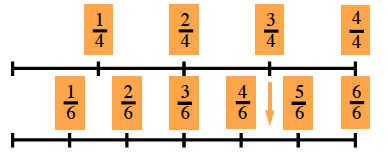### Home > CC2 > Chapter 3 > Lesson 3.3.1 > Problem3-94

3-94.

Use any method to divide.

1. $\frac{3}{4}\div\frac{1}{6}$

Here is an example of a linear model.1. $\frac{5}{12}\div(-\frac{1}{6})$

The common denominator is $12$.

$\frac{5}{12}\div\left(-\frac{2}{12}\right)=5\div-2=-\frac{5}{2}=-2\frac{1}{2}$

1. $-\frac{2}{3}\div\frac{1}{2}$

What Giant One should you multiply by?

1. $-2\frac{2}{3}\div(-\frac{1}{2})$

Use one of the methods shown in parts (a), (b) or (c).

$\frac{16}{3}=5\frac{1}{3}$